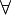# 12-240/Classnotes for Tuesday October 2

The "vitamins" slide we viewed today is here.

Today, the professor introduces more about subspace, linear combination, and related subjects.

## Subspace

Remind about the theorem of subspace: a non-empty subset W ⊂ V is a subspace iff is is closed under the operations of V and contains 0 of V

Proof:

First direction "=>":

if a non-empty subset W ⊂ V is a subspace , then W is a vector space over the operations of V .

=> + W is closed under the operations of V.

+ W has a unique identity of addition:$\forall\!\,$ a$\in\!\,$ W: 0 + a = a

Moreover, a a$\in\!\,$ V. Hence 0 is also identity of addtition of V

Second direction "<=":

if a non-empty subset W ⊂ V is closed under the operations of V and contains 0 of V

we need to prove that W is a vector space over operations of V, hence, and subspace of V.

Namely, we need to show that W satisfies all axioms of a vector space, but now we just consider some axioms and leave the rest to readers.

VS1: Consider$\forall\!\,$ x,y$\in\!\,$ W => a,b$\in\!\,$ V

While V is a vector space

thus x + y = y + x ( and the sum$\in\!\,$ W since W is closed under addition)

VS2: (x + y) + z = x + (y + z) is proven similarly

VS3: As given, 0 of V$\in\!\,$ W, pick any a in W ( possible since W is not empty)

So, a$\in\!\,$ V hence a + 0 = a

Thus 0 is also additive identity element of W